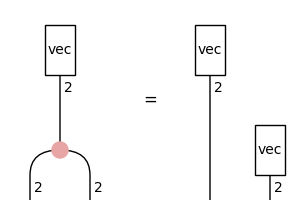# Spider#

class discopy.tensor.Spider(*args, **kwargs)[source]#

A tensor spider is a frobenius spider in a tensor diagram.

Parameters:
• n_legs_in (int) – The number of legs in.

• n_legs_out (int) – The number of legs out.

• typ (Dim) – The dimension of the spider.

• data – The phase of the spider.

Examples

```>>> vector = Box('vec', Dim(1), Dim(2), [0, 1])
>>> spider = Spider(1, 2, Dim(2))
>>> assert (vector >> spider).eval() == (vector @ vector).eval()
>>> from discopy.drawing import Equation
>>> Equation(vector >> spider, vector @ vector).draw(
...     path='docs/_static/tensor/frobenius-example.png', figsize=(3, 2))
```